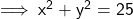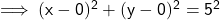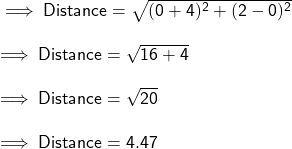## does the point (-4, 2) lie inside or outside or on the circle x^2 + y^2 = 25?​ ​

Question

does the point (-4, 2) lie inside or outside or on the circle x^2 + y^2 = 25?​

in progress 0
2 months 2021-07-30T15:53:27+00:00 2 Answers 6 views 0

1. Given equation of the Circle is ,And we need to tell that whether the point (-4,2) lies inside or outside the circle. On converting the equation into Standard form and determinimg the centre of the circle as ,Here we can say that ,

• Centre = (0,0)

Finding distance between the two points :-Here we can see that the distance of point from centre is less than the radius.

Hence the point lies within the circle.